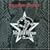Forum

(GLQuake) (Semi-Tutorial) Basic Surface Batcher

Post tutorials on how to do certain tasks within game or engine code here.

(GLQuake) (Semi-Tutorial) Basic Surface Batcher

Draw call batching is key to performance; as polycounts go up, getting some good batching in will see less of a performance impact. Sometimes it's quite dramatic: maps that previously plummeted to 20fps can stay in the hundreds; sometimes less so: simpler maps don't suffer from as much draw call overhead, so the gains are more marginal.

This is a simple (and simplistic) surface batcher that works with GLQuake; it's almost drop-n-go, and uses vertex arrays to achieve at least some degree of batching. It's far from being perfect, and there is plenty of room for optimization - ideally you'd set up your vertex data so that you can make glVertexPointer and glTexCoordPointer calls directly to the data itself rather than having to copy vertexes around.

It can also be used as a jumping-off point for a VBO implementation, but I haven't gone that far with this code - for this one I've decided to stick with GL 1.1 calls.

One limitation is that it will not work with multitexturing. It wouldn't have been too difficult, but the old GL_SGIS_multitexture extension that GLQuake uses doesn't support vertex arrays. Ideally everyone using multitexturing would jump to GL_ARB_multitexture, which does.

Code: Select all
`// these could go in a vertexbuffer/indexbuffer pair#define MAX_BATCHED_SURFVERTEXES   65535#define MAX_BATCHED_SURFINDEXES      262144const int sizeofvertex = VERTEXSIZE * sizeof (float);float r_batchedsurfvertexes[MAX_BATCHED_SURFVERTEXES * VERTEXSIZE];unsigned short r_batchedsurfindexes[MAX_BATCHED_SURFINDEXES];int r_numsurfvertexes = 0;int r_numsurfindexes = 0;void R_BeginBatchingSurfaces (int texcoordindex){   glEnableClientState (GL_VERTEX_ARRAY);   glVertexPointer (3, GL_FLOAT, sizeofvertex, &r_batchedsurfvertexes);   glEnableClientState (GL_TEXTURE_COORD_ARRAY);   glTexCoordPointer (2, GL_FLOAT, sizeofvertex, &r_batchedsurfvertexes[texcoordindex]);   r_numsurfvertexes = 0;   r_numsurfindexes = 0;}void R_EndBatchingSurfaces (void){   if (r_numsurfvertexes && r_numsurfindexes)   {      glDrawElements (GL_TRIANGLES, r_numsurfindexes, GL_UNSIGNED_SHORT, r_batchedsurfindexes);   }   r_numsurfvertexes = 0;   r_numsurfindexes = 0;}void R_BatchSurface (glpoly_t *p){   int i;   int numindexes = (p->numverts - 2) * 3;   unsigned short *ndx;   if (r_numsurfvertexes + p->numverts >= MAX_BATCHED_SURFVERTEXES) R_EndBatchingSurfaces ();   if (r_numsurfindexes + numindexes >= MAX_BATCHED_SURFINDEXES) R_EndBatchingSurfaces ();   memcpy (&r_batchedsurfvertexes[r_numsurfvertexes * VERTEXSIZE], p->verts, p->numverts * sizeofvertex);   ndx = &r_batchedsurfindexes[r_numsurfindexes];   for (i = 2; i < p->numverts; i++, ndx += 3)   {      ndx = r_numsurfvertexes;      ndx = r_numsurfvertexes + i - 1;      ndx = r_numsurfvertexes + i;   }   r_numsurfvertexes += p->numverts;   r_numsurfindexes += numindexes;}`

To implement this, first you call R_BeginBatchingSurfaces before you start drawing surfaces. Give it the index of the texcoord set you're going to use, which will be 3 for world textures or 5 for lightmaps.

Then, for each and every state or texture change you make, you need to call R_EndBatchingSurfaces. This is important - a state change requires you to break the current batch and begin a new one (R_EndBatchingSurfaces will do both of these steps). This is another reason to not implement this on GLQuake's multitexture path, by the way - the amount of state and texture changes made is phenomenal and you'll get little batching going on. You need some proper sorting first, which GLQuake's single-textured path will do for you.

Then for each surf, instead of going through the glBegin/glEnd dance, just call R_BatchSurface.

Finally, when you're done with drawing surfaces (e.g. at the end of each texture in DrawTextureChains or each lightmap in R_BlendLightmaps) also call R_EndBatchingSurfaces.

Note that I don't bother shutting down the vertex arrays here - with GLQuake that's not necessary, but an engine that uses arrays elsewhere will need to. At the last call to R_EndBatchingSurfaces would be a good place to put that.

It needs to be restated again that simple content will not exhibit as much gain, so if you benchmark this with timedemo demo1, demo2 or demo3 you're likely to be mildly disappointed. You need to throw something heavier at it - try marcher, Masque of the Red Death or something like that for best results.